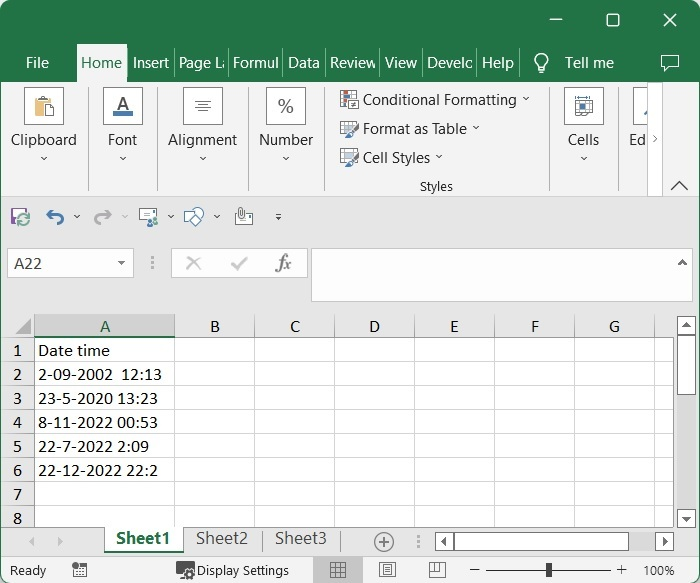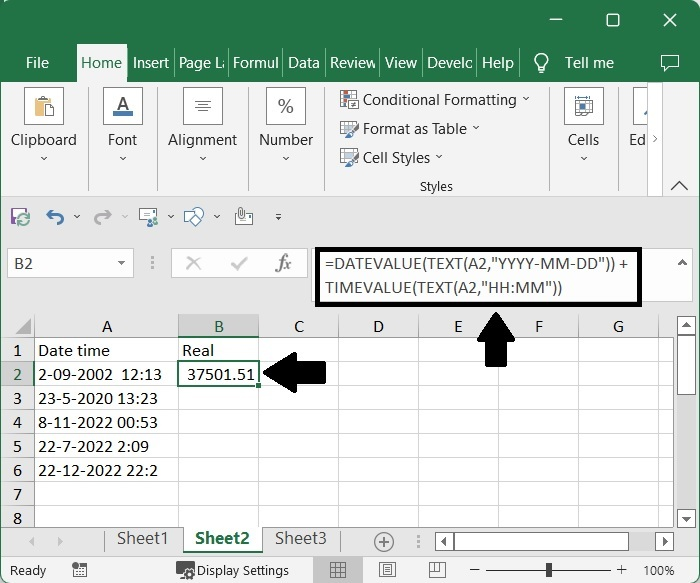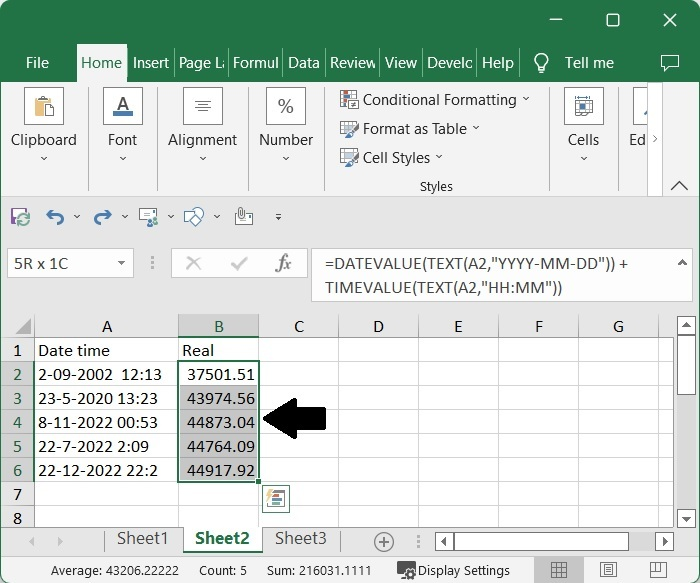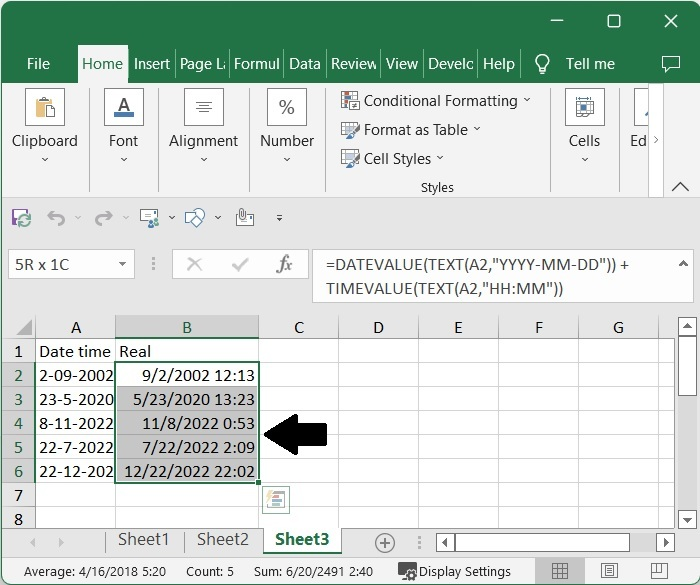# How to Convert Text Datetime Format to Real Datetime Format in Excel?

Sometimes in Excel, you can see that the date and time are given in the form of strings, and you will not be able to perform operations related to data and time with those values. So, it is very important for us to convert these kinds of formats into the correct format. If we try to do this manually, it can be time-consuming and sometimes inaccurate. We can complete this task using the formulas to get more accurate results and save a lot of time.

Read this tutorial to learn how you can convert text date and time formats to real date and time formats in Excel. The original format that is used to represent data time is known as "real date time" format. We finish this task in Excel by using the DATEVALUE and TIME VALUE functions.

## Converting Text Datetime Format to Real Datetime Format in Excel

In this case, we'll use the DATEVALUE and TIMEVALUE formulas to get one result, and then use the autofill handle to get all of the other results. Let's look at a simple procedure for converting seconds to time in Excel.

### Step 1

Consider the following image of an excel sheet that contains datetime formatted information for text values.Now, in cell B2, click on an empty cell and enter the formula as follows −

=DATEVALUE(TEXT(A2,"YYYY-MM-DD"))+TIMEVALUE(TEXT(A2,"HH:MM")) and click Enter to get our first result, which looks similar to the below image. In the formula, A2 is the address of the cell where our value is present on the sheet.

Empty cell > Formula > Enter### Step 2

Then drag down from the first result using the auto-fill handle to get all the results similar to the below image.### Step 3

Now we need to format the cells to complete our task. To do so, select the values, then right-click and select format cells, click on "Custom," enter the type as m/d/yyyy h:mm, select the date and time formats, and click OK.

Select data > Right click > Format cell > Custom > m/d/yyyy h:mm > OK## Conclusion

In this tutorial, we used a simple example to demonstrate how you can convert text datetime format to real data time format in Excel.